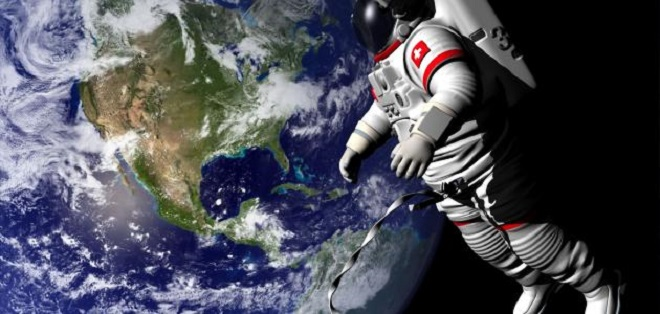# Where is the Earth's Center of Gravity Located?

The center of gravity of the Earth-Moon system actually lies within the Earth's body; indeed, it is about 42 km away from the center of the earth in the direction of the moon and 1700 km below the surface of the earth.Where is the Earth's Center of Gravity?

## What is the Center of Gravity?

The center of gravity can be defined physically as an imaginary point in place suitable for some computational procedures.

The center of gravity is a geometric feature of an object. It is the average location of the weight of an object.

In some cases, the center of gravity is calculated when designing fixed structures such as buildings and bridges, or when trying to predict the behavior of a moving object when it is affected by the Earth's gravity.

It is possible to know the center of gravity when the gravitational field is uniform by knowing the center of mass of the body since the center of mass of the body is identical to the center of gravity in this case.

Earth's gravity can also be defined as a force that pulls material down towards the center of the earth, and based on the concept of Earth's gravity, whatever is thrown up will drop another down, but gravity does not always work according to this concept because balance plays a role in influencing it, in relation to the material’s center of gravity, which directly depends on the shape of the material.

### Where is Earth's Center of Gravity?

The center of gravity of the Earth-Moon system actually lies within the Earth's body; indeed, it is about 42 km away from the center of the earth in the direction of the moon and 1700 km below the surface of the earth.

Scientifically, the center of gravity of the Earth can be defined in two ways: either it is the center of mass of the solid earth, or it is the center of mass of the entire global system, including its solid ground, ice sheets, oceans, and the atmosphere. However, the center of the globe is not scientifically determined in either case, as the Earth is constantly changing due to changing tectonic forces and climate forces.

#### Does the Universe Have a Center of Gravity?

There is no center of gravity for the universe, meaning that it does not have a point of origin, and this can be understood by referring to the Big Bang theory about 13.7 billion years ago, which states that the universe did not explode from a central point, as the universe began to be small in size and then every point in the universe began to expand equally, and this expansion is still continuing.

It is worth noting that scientists are still not sure of the validity of its limitations, as the scientist Ryden said that “due to the limited distance traveled by light since the Big Bang, the observations of cosmologists provide only a finite glimpse of the cosmos, but the entire universe may be infinite."

1.